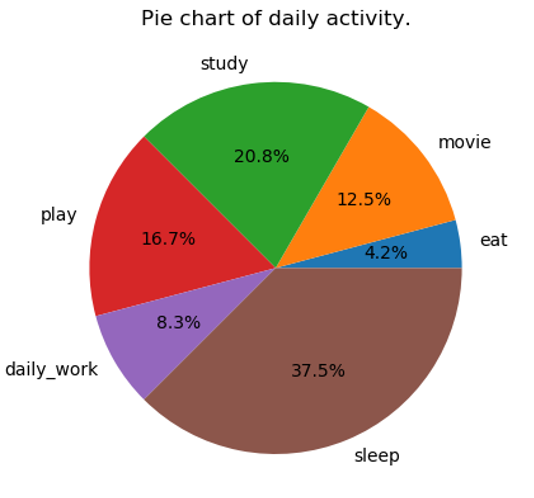# Draw a pie chart that shows our daily activity in Python

Here, we will see how to draw a pie chart that shows our daily activity in the Python programming language?
Submitted by Bipin Kumar, on November 28, 2019

The pie chart represents the quantity in percentages, and the total sum of all the segments of the pie chart must be equal to 100%. It is used to visualize the given data in percentage. The user will provide us one list of daily activities and another list that shows the time taken daily to do each activity and by using these lists we have to draw a pie chart by using the Python. One thing will come to your mind that to draw a pie chart of daily activity, initially, we have to calculate the percentage of each activity time then draw the pie chart and it will take a lot of time. Yes, this approach definitely will take a lot of time and that's why we will not go for this approach. So, don't worry about it because Python provides us an in-built matplotlib library which makes it so much easier. Before using the matplotlib library in the program, we will see a little bit about it and the installation process.

matplotlib library is one of the most useful libraries of Python. It is used for visualization of given data in 2D plots. By using this, we can draw plots, pie charts, histograms, scatterplots, etc.

Algorithm to solve this problem

1. Import pyplot module from the matplotlib library as plt.
2. Take the daily activity and time taken to do the activity in two arrays.
3. Now, draw the pie chart with the title 'Pie chart of daily activity'.

So, let's start writing a few lines of code in Python by implementing the above algorithm in a simple way.

Program:

```import matplotlib.pyplot as plt

A=['eat', 'movie', 'study', 'play','daily_work','sleep']
T=[1,3,5,4,2,9]

plt.pie(T, labels=A,autopct= '%1.1f%%')
plt.title('Pie chart of daily activity.')
plt.show()
```

OutputPreparation